Our World Statistics Day conversations have been a great reminder of how much statistics can inform our lives. Do you have an example of how statistics has made a difference in your life? Share your story with the Community!
Choose Language Hide Translation Bar
Functional programming using JSL objects

At the end of How to use Define Class I hinted there was something interesting about JSL class instances being hard to copy. if X refers to an instance, Y=X makes both Y and X refer to the same instance. The same thing happens when you pass an instance to a user defined function: the function's value is not a copy of the instance; it refers to the same instance. So changing the instance inside the function changes it for the caller as well. If you have ever passed a matrix to a function and modified it in the function, expecting the caller's copy to be updated, you know JSL passes arguments to user functions by value.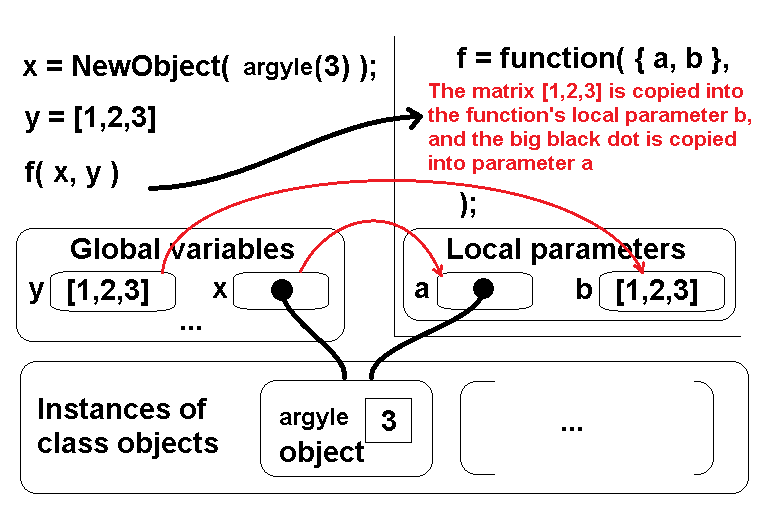JMP passes arguments by making copies, but...

Notice the matrix is copied, so changes to the matrix inside the function are made to the copy, not the original. Notice the big black reference dot is also copied, but not the object it refers to. This causes beginning JAVA programmers many headaches because they have not seen this picture. Within the function, you can do two very different things to variable a.

1. you can call a method, like a:set(42);
2. you can assign a value, like a=42;

In case (1) you change the argyle object's value from 3 to 42. This is probably what you mean to do. in case (2), you overwrite the big black dot next to a with 42. You probably didn't mean to do that.

The following code uses objects that keep references to other objects. All of the objects have a next() method, so the objects can be composed in interesting ways. The next() method produces the next value in a sequence of values. Some objects represent a constant and always produce the same next value. Others, like the add object, have two other objects (the left side and right side of the + operator). Add:next() calls left:next() and right:next() and adds the two answers.

The complete JSL is attached. Here it is with the pictures interleaved. Let's start with the simplest class.

``````// number/matrix wrapper class
Define Class(
"vwrap",
m_v = .; // holds the number or matrix
_init_ = Method( {v},
If( Type( v ) == "Class",
Throw( "vwrap is for number or matrix, not class " || Char( v << getname ) )
);
m_v = v;
);
set = Method( {v},
If( Type( v ) == "Class",
Throw( "vwrap is for number or matrix, not class " || Char( v << getname ) )
);
m_v = v;
);
next = Method( {},
m_v; // return
);
);``````

Vwrap is the name of the class, and it wraps a value, either a number or a matrix. It has a setter that will reject values it doesn't appreciate and a getter, named next(), to return the next value in a sequence. Because it is a bit ugly to write NewObject(Vwrap(42)), let's also make a helper function to make Vwraps.

``````// helper function v(...) converts a scalar or matrix to a vwrap, or returns an instance unchanged
v = Function( {x},
If( Type( x ) == "Class",
If( !(x << Contains( "next" )),
Throw( "v expects number, matrix, or one of the classes it supports, not class " || (x << getname) )
);
x; // x supports a next function, it is probably OK
, // else
If( !Is Number( x ) & !Is Matrix( x ),
Throw( "v expects number or matrix, not " || Type( x ) )
);
New Object( vwrap( x ) ); // wrap the number or matrix
)
);``````

v(...) will get called a lot, sometimes with a class instance, sometimes with a scalar or matrix, and it needs to return the class instance unchanged when it gets one. It might as well check that there is a next() in the class at the same time, just in case there are other classes that might get mixed up.

Next, a largely boiler plate function to make a graph.

``````// global function gr(...) makes a graph
gr = Function( {Xvar, Yvar, title, n},
{Xs, Ys, dt}, 	// locals
Yvar = v( Yvar );
Xvar = v( Xvar );
Ys = J( n, 1, Yvar:next() );
Xs = J( n, 1, Xvar:next() );
dt = As Table( Ys || Xs, <<invisible, <<ColumnNames( {"y", "x"} ) );
Eval(// capture the invisible dt as a constant in onclose(), below
Eval Expr(
dt << Graph Builder(
title( title ),
Show Control Panel( 0 ),
Show Legend( 0 ),
Variables( X( :x ), Y( :y ) ),
Elements( Line( X, Y, Legend( 2 ), Row order( 1 ) ), Points( X, Y, Legend( 3 ) ) ),

SendToReport(
Dispatch( {}, "x", ScaleBox, {Add Ref Line( 0, "Solid", "Black", "", 1 ), Label Row( Show Major Grid( 1 ) )} ),
Dispatch( {}, "y", ScaleBox, {Add Ref Line( 0, "Solid", "Black", "", 1 ), Label Row( Show Major Grid( 1 ) )} ),
Dispatch( {}, title, OutlineBox, {Set Title( "" )/*, Image Export Display( Normal )*/} ),
Dispatch( {}, "graph title", TextEditBox, {Set Text( title )} )
),

<<onclose(
Close( Expr( dt ), nosave );
1;
)
)
)
);
);``````

You can see the calls to v() for the X and Y parameters; those can be simple numbers that will get wrapped, or other classes with a next() method. Then the J() function makes matrices of N values, and a invisible table is made, and Graph Builder runs, with a bit of magic to close the hidden table when it is no longer needed. Let's try it.

``gr( 13, 42, "Value 13,42", 1 );``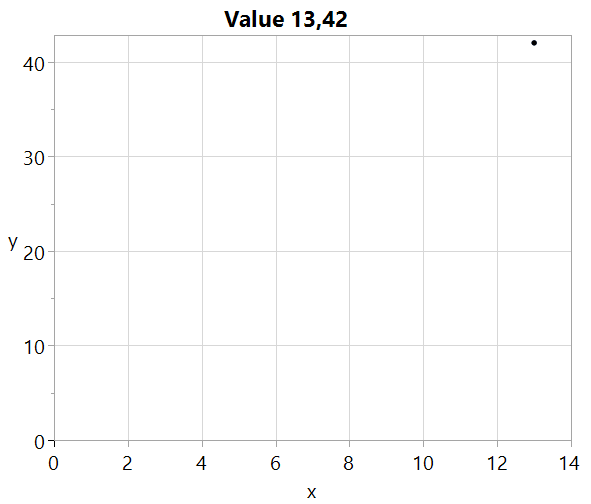A single point, at x=13, y=42

Hang on, it will get better...Let's make a counter class that will return 0,1,2,3,... on successive calls to next().

``````// counter class
Define Class(
"counter",
m_rate = .; // non zero increment
m_pos = empty(); // internal position
_init_ = Method( {rate},
m_rate = v( rate );
);
next = Method( {},
rate = m_rate:next();
// see peek() comment in "sin" class
If( Is Empty( m_pos ),
result = 0 * rate; // get scalar or matrix dimension on first call
m_pos = rate; // for next time
, // else
result = m_pos; // from last tim
m_pos += rate; // for next time
);
result; // return
);
);``````

The _init_ method takes a rate that determines how fast the counter increments. Rate can be a constant, typically 1, or it can be another class instance. Here's a helper function to make a counter, and a first graph.

``````// global function to make a counter
ctr = Function( {rate = 1},
New Object( counter( rate ) )
);

gr( ctr(),  ctr() , "count up A (equal steps)", 5 );``````

Notice two separate counter instances were passed to gr(...) for the X and Y axes.Two counters, running in parallel, for five steps starting at zero.

Now, for something different: let's make the counter's rate be another counter.

``````// this variation begins to show the power of composition
gr( ctr(), ctr( ctr() ), "count up B (first step is 0)", 5 );``````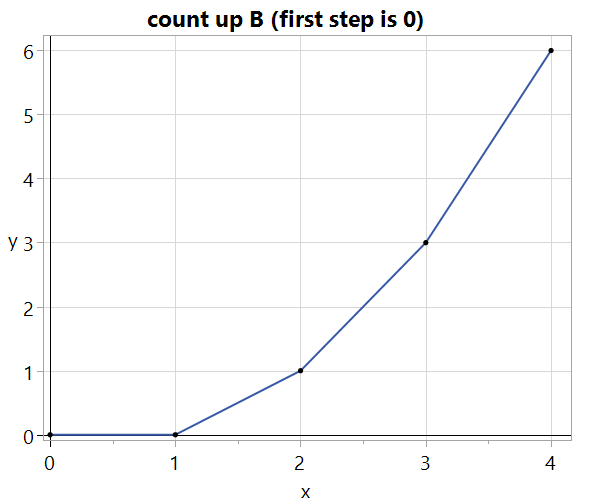The first step is zero because the rate counter's first result is zero.

This graph's Y axis is using functional composition of two counters. Lets add a sin() class.

``````// sin() wrapper class
Define Class(
"sin",
m_rate = .; // 0<step<PI.
m_amp = .; // amplitude
m_pos = .; // internal position
_init_ = Method( {rate, amp}, //
m_rate = v( rate );
m_amp = v( amp );
// pos needs same dimension (or scalar) as rate, but start at 0.
// This is going to use up the first value from rate prematurely
// and could be rearranged like the counter class to avoid that,
// but you can't really see the issue in the variable frequency
// graph. I think adding a peek() might be a good choice.
m_pos = -m_rate:next();
);
next = Method( {},
// amp and rate can mix'n'match scalar and matrix
m_amp:next() :* Sin( m_pos += m_rate:next() ) // return
);
);``````

Sin produces an infinite sine wave at a frequency and amplitude determined by constants or other class instances. It also keeps an internal position. You can tell from the comments that it might need more thought, but it works for this demo. Here's a single cycle waveform.

``gr( ctr(), New Object( Sin( 2 * Pi() / 100, 3 ) ), "sin()", 101 );``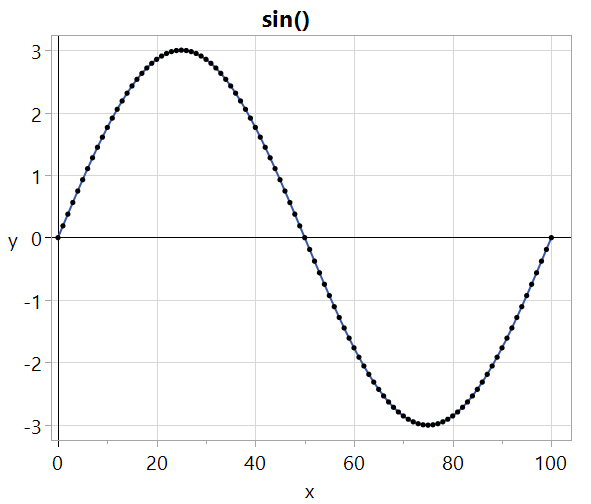Single cycle of sine wave

Similar to the counter with an increment of another counter, a sine wave can have a variable frequency.

``gr( ctr(), New Object( Sin( New Object( Sin( .0002, 1 ) ), 3 ) ), "variable frequency", 1000 );``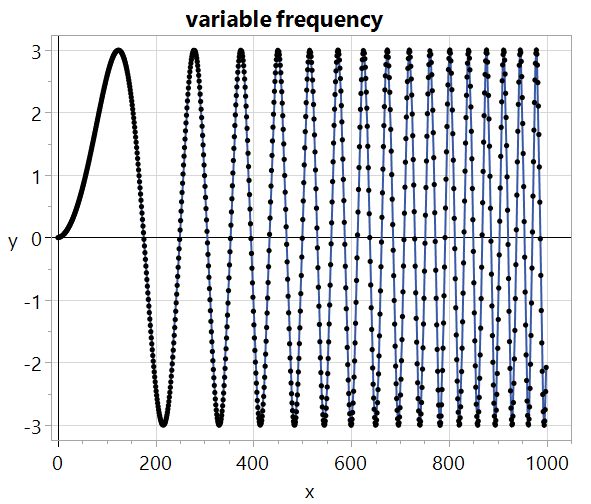Ramping up the frequency using the first bit of another sine wave

And two different frequency sine waves can be plotted against each other.

``gr( New Object( Sin( 4 * Pi() / 99, 3 ) ), New Object( Sin( 6 * Pi() / 99, 3 ) ), "2X3 sin()", 100 );``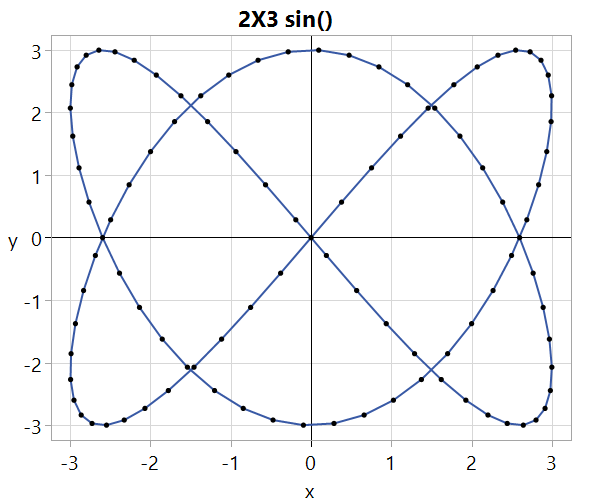Simple integer ratios make nice patterns

Another class and helper function; the class adds one or more other classes together and the offset helper adds a constant...or another function, probably...untested.

``````// add class
Define Class(
m_a = {};
//m_b = .;
_init_ = Method( {a, b = Empty()},
If( Is Empty( b ),
For( i = 1, i <= N Items( a ), i += 1,
Insert Into( m_a, v( a[i] ) )
),
m_a = v( a );
m_a = v( b );
)
);
next = Method( {},
result = m_a:next();
For( i = 2, i <= N Items( m_a ), i += 1,
result = result + m_a[i]:next()
);
result;
);
);

// helper function uses add to offset another value by off
offset = function({off,x},
);``````

With the offset helper one of the early graphs that had an initial zero step can be reworked to have an initial step of one.

``````// compared to a previous example, this one increments earlier
gr( ctr(), ctr( offset(1,ctr( 1 )) ), "count up C (first step is 1)", 5 );``````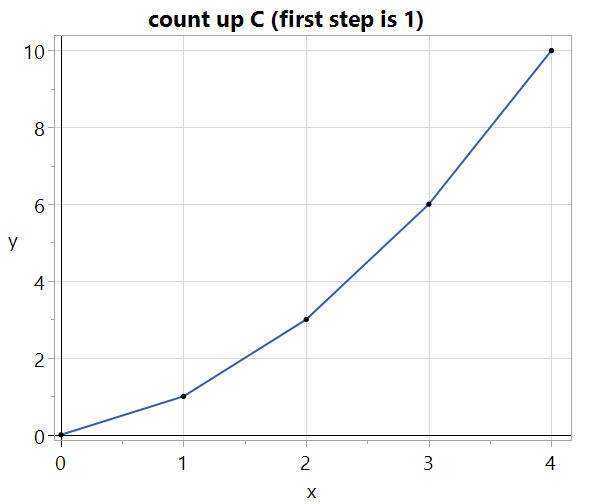Now all the steps are one bigger than before.

``````// building a square wave by summing odd harmonics
n = 100;
basefreq = 2 * Pi() / n;
freq1 = New Object( Sin( 1 * basefreq, 1 / 1 ) );
freq2 = New Object( Sin( 3 * basefreq, 1 / 3 ) );
freq3 = New Object( Sin( 5 * basefreq, 1 / 5 ) );
freq4 = New Object( Sin( 7 * basefreq, 1 / 7 ) );
sum = New Object( Add( {freq1, freq2, freq3, freq4} ) );
gr( ctr(), sum, "4 Odd Harmonics", n + 1 );``````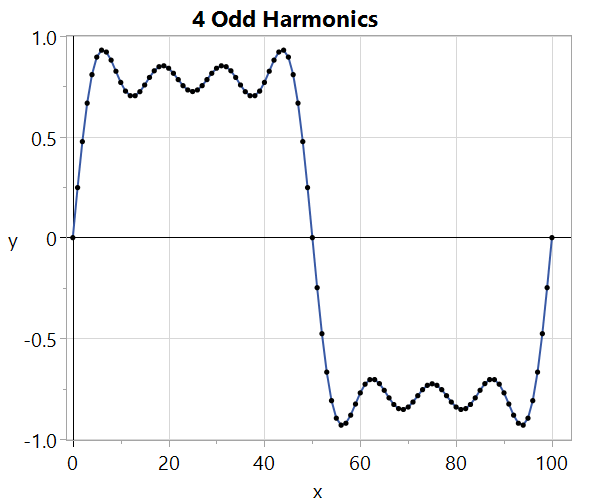Summing odd harmonics at reduced amplitude approximates a square wave.

``````// same square wave, a lot more odd harmonics
n = 200;
basefreq = 2 * Pi() / n;
freqs = {};
For( i = 1, i <= 23, i += 2,
Insert Into( freqs, New Object( Sin( i * basefreq, 1 / i ) ) )
);
gr( ctr(), New Object( Add( freqs ) ), "12 Odd Harmonics", n + 1 );``````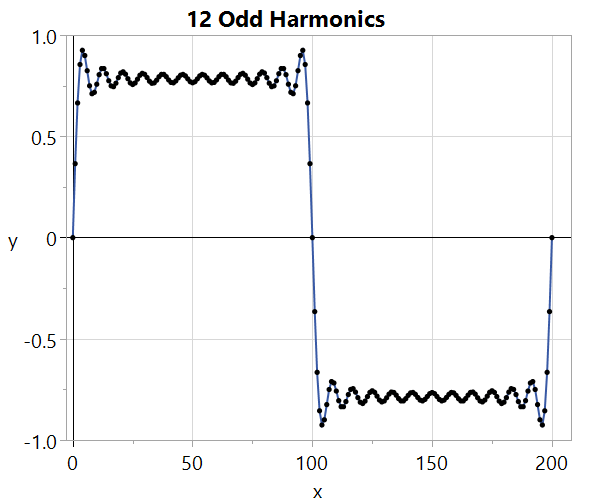More harmonics help square it up

``````// multiply class
Define Class(
"multiply",
m_a = {};
_init_ = Method( {a, b = Empty()},
If( Is Empty( b ),
For( i = 1, i <= N Items( a ), i += 1,
Insert Into( m_a, v( a[i] ) )
),
m_a = v( a );
m_a = v( b );
)
);
next = Method( {},
result = m_a:next();
For( i = 2, i <= N Items( m_a ), i += 1,
result = result :* m_a[i]:next()
);
result;
);
);

// multiply two waveforms
n = 1000;
freq1 = New Object( Sin( .1, 1 ) );
freq2 = New Object( Sin( 2 * Pi() / n, 1 ) );
prod = New Object( Multiply( freq1, freq2 ) );
gr( ctr(), prod, "multiply", n + 1 );``````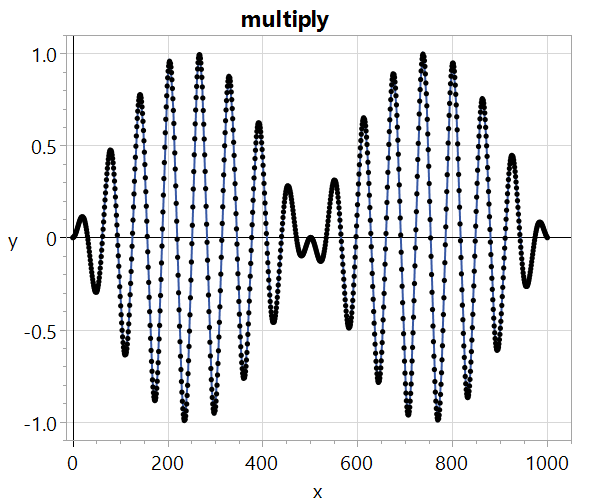Multiply two frequencies; the lower frequency creates the envelope.

``````// similar, but control amplitude of freq1 using another sin wave
n = 1000;
freq2 = New Object( Sin( 2 * Pi() / n, 1 ) ); // lo freq
freq1 = New Object( Sin( .1, freq2 ) ); // hi freq, control amplitude with freq2
gr( ctr(), freq1, "amp is sin", n + 1 );``````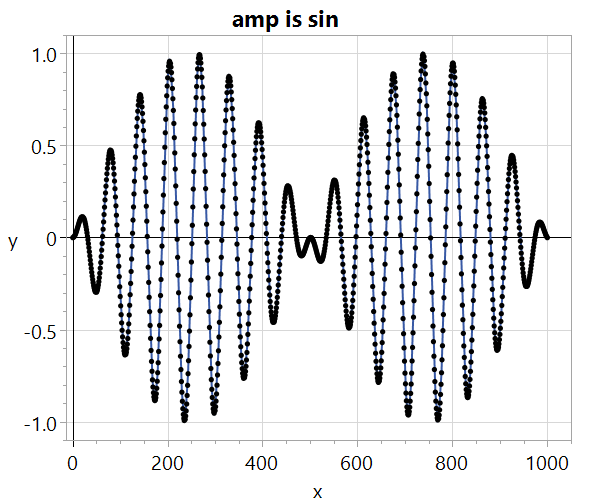Alternatively, control the amplitude of the higher frequency using the lower frequency.

Finally, make a vwrap holding a matrix of odd harmonic frequencies and make a new class to sum the matrix into a scalar.

``````// try a matrix...use v(...) to make a vwrap to hold the matrix
n = 200;
mat = v( ((1 :: 49 :: 2) * (2 * Pi() / n)) );
// add the sin wrapper. sinmat:next() returns a row vector of 5 sin values
sinmat = New Object( Sin( mat, 1 / (1 :: 49 :: 2) ) );

// matrixSum assumes the argument class is returning a matrix and returns the scalar sum
Define Class(
"matrixSum",
m_mat = .;
_init_ = Method( {mat},
m_mat = v( mat )
);
next = Method( {},
Sum( m_mat:next() )
);
);

gr( ctr(), New Object( matrixSum( sinmat ) ), "25 Odd Harmonics", n + 1 );``````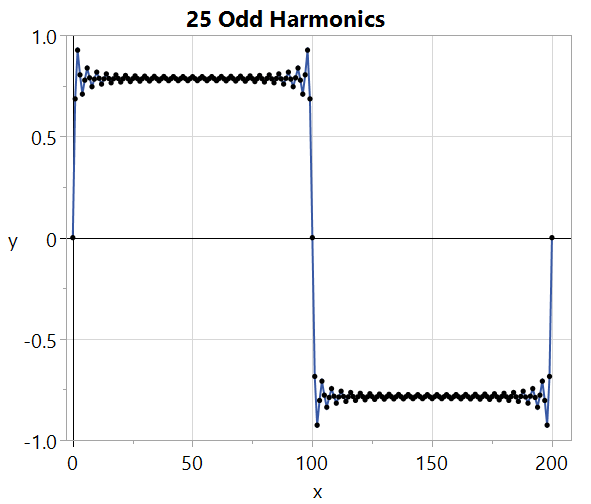It will take a lot more harmonics to really reduce the over-shoot and the ripples.

Article Labels

There are no labels assigned to this post.

Article Tags